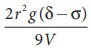Home | | Physics 11th std | Viscosity of a Liquid By StokeтАЩs Method

# Viscosity of a Liquid By StokeтАЩs Method

To determine the co-efficient of viscosity of the given liquid by stokeтАЩs method

VISCOSITY OF A LIQUID BY STOKEтАЩS METHOD

## AIM

To determine the co-efficient of viscosity of the given liquid by stokeтАЩs method

## APPARATUS REQUIRED

A long cylindrical glass jar, highly viscous liquid, metre scale, spherical ball, stop clock, thread.

## FORMULAWhere

╬╖ - Coefficient of viscosity of liquid (N s mтАУ2)

r тЖТ radius of spherical ball ( m )

╬┤тЖТ density of the steel sphere ( kg mтАУ3 )

╧ГтЖТ density of the liquid ( kg mтАУ3 )

g тЖТ acceleration due to gravity (9.8 m sтАУ2 )

V тЖТ mean terminal velocity ( m sтАУ1 )

## DIAGRAM## PROCEDURE

┬╖         A long cylindrical glass jar with markings is taken.

┬╖         Fill the glass jar with the given experimental liquid.

┬╖         Two points A and B are marked on the jar. The mark A is made well below the surface of the liquid so that when the ball reaches A it would have acquired terminal velocity V.

┬╖         The radius of the metal spherical ball is determined using screw gauge.

┬╖         The spherical ball is dropped gently into the liquid.

┬╖         Start the stop clock when the ball crosses the point A. Stop the clock when the ball reaches B.

┬╖         Note the distance between A and B and use it to calculate terminal velocity.

┬╖         Now repeat the experiment for different distances between A and B. Make sure that the point A is below the terminal stage.

## OBSERVATIONS

To find Terminal Velocity:## CALCULATION

Density of the spherical ball ╬┤ = ________ kg mтИТ3

Density of the given liquid ╧Г = ________ kg mтИТ3

Coefficient of viscosity of the liquid ╬╖ = 2r2g(╬┤ тИТ╧Г) / 9V  == ________ N s mтАУ2

## RESULT

The coefficient of viscosity of the given liquid by stokeтАЩs method ╬╖ = ________ NsmтАУ2

Tags : Physics Laboratory Practical Experiment , 11th Physics : Practical Experiment
Study Material, Lecturing Notes, Assignment, Reference, Wiki description explanation, brief detail
11th Physics : Practical Experiment : Viscosity of a Liquid By StokeтАЩs Method | Physics Laboratory Practical Experiment